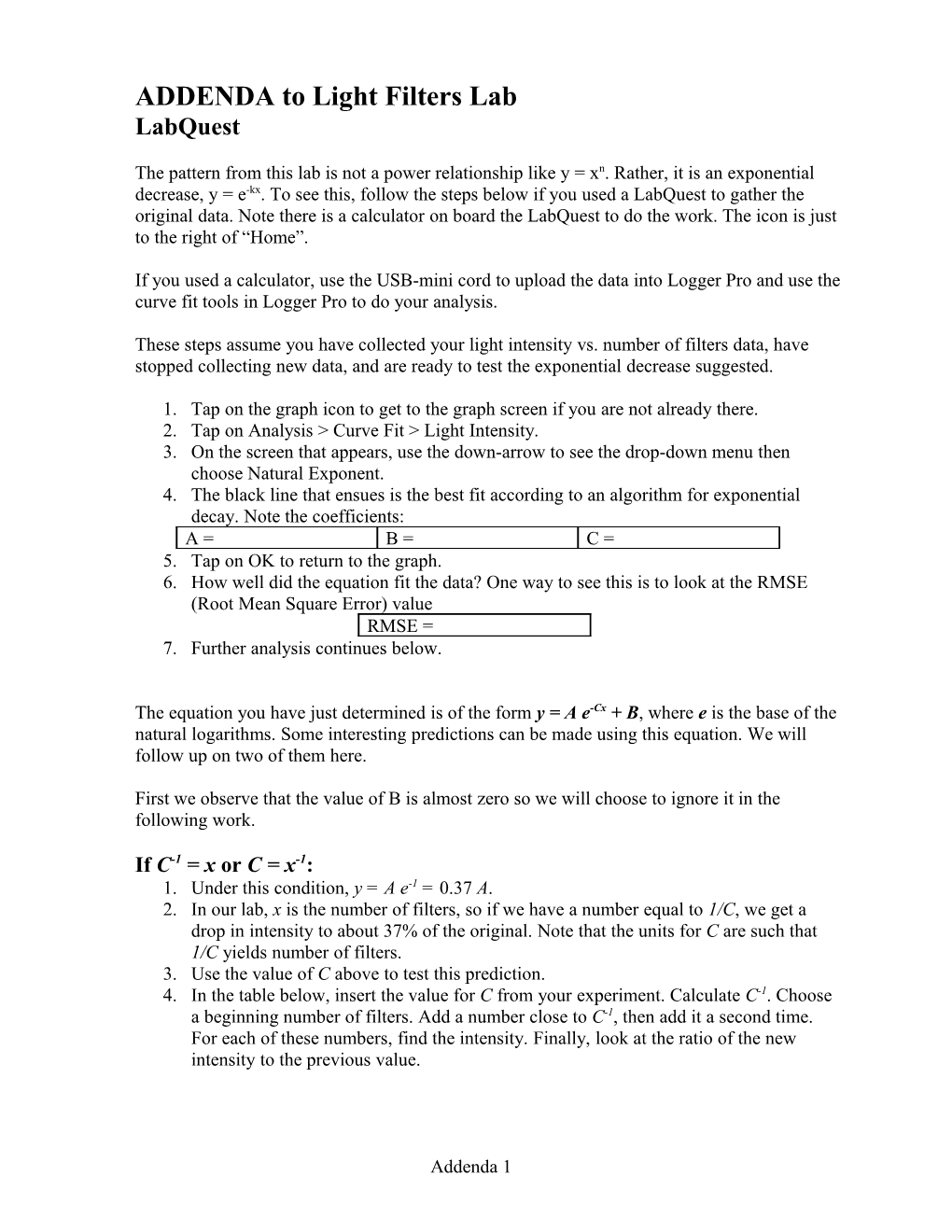# ADDENDA to Light Filters Lab## ADDENDA to Light Filters Lab

### LabQuest

The pattern from this lab is not a power relationship like y = xn. Rather, it is an exponential decrease, y = e-kx. To see this, follow the steps below if you used a LabQuest to gather the original data. Note there is a calculator on board the LabQuest to do the work. The icon is just to the right of “Home”.

If you used a calculator, use the USB-mini cord to upload the data into Logger Pro and use the curve fit tools in Logger Pro to do your analysis.

These steps assume you have collected your light intensity vs. number of filters data, have stopped collecting new data, and are ready to test the exponential decrease suggested.

1. Tap on the graph icon to get to the graph screen if you are not already there.
2. Tap on Analysis > Curve Fit > Light Intensity.
3. On the screen that appears, use the down-arrow to see the drop-down menu then choose Natural Exponent.
4. The black line that ensues is the best fit according to an algorithm for exponential decay. Note the coefficients:

A = / B = / C =
2. How well did the equation fit the data? One way to see this is to look at the RMSE (Root Mean Square Error) value

RMSE =
1. Further analysis continues below.

The equation you have just determined is of the form y = A e-Cx + B, where e is the base of the natural logarithms. Some interesting predictions can be made using this equation.We will follow up on two of them here.

First we observe that the value of B is almost zero so we will choose to ignore it in the following work.

If C-1 = x or C = x-1:

1. Under this condition, y = A e-1 = 0.37 A.
2. In our lab, x is the number of filters, so if we have a number equal to 1/C, we get a drop in intensity to about 37% of the original. Note that the units for C are such that 1/C yields number of filters.
3. Use the value of C above to test this prediction.
4. In the table below, insert the value for C from your experiment. Calculate C-1. Choose a beginning number of filters. Add a number close to C-1, then add it a second time. For each of these numbers, find the intensity. Finally, look at the ratio of the new intensity to the previous value.

C =
C-1 =
No. of Filters / Light Intensity
1: / 1: /

#### Ratio

2: / 2: / 2/1:
3: / 3: / 3/2:

Half Brightness:

1. If we let y = A/2, the value A will have dropped to half. The condition under which this is true is:

y = A/2 = A e-Cx

1/2 = e-Cx … now take the natural logarithm (ln) of both sides

-0.693 = -Cx

x1/2 = 0.693/C … note the use of the subscript

1. There is a number of filters equal to 0.693/C such that the amount of light intensity drops to half its former value after we add them. The same number more drops it to half that and so on.
2. Test this by calculating the value of x1/2 and testing some values from your data table.

x2 = x1 + x1/2, etc.

C = / x1/2 =
No. of Filters / Light Intensity
x1 / I1
x2 / I2
x3 / I3
1. Doyou see a parallel between this experiment and a value that’s often talked about when discussing radiation?

C. Bakken

October 2008